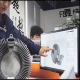# Differential Input Single-Ended Output Amplifier Simple Solution

Many applications require the use of low-power, high-performance differential amplifiers to convert small differential signals into readable ground-referenced output signals. The two inputs usually share a large common-mode voltage. The differential amplifier rejects the common-mode voltage, and the residual voltage, after being amplified, appears as a single-ended voltage at the amplifier output. The common-mode voltage can be either AC or DC and is usually greater than the differential input voltage.

Many applications require the use of low-power, high-performance differential amplifiers to convert small differential signals into readable ground-referenced output signals. The two inputs usually share a large common-mode voltage. The differential amplifier rejects the common-mode voltage, and the residual voltage, after being amplified, appears as a single-ended voltage at the amplifier output. The common-mode voltage can be either AC or DC and is usually greater than the differential input voltage. The rejection effect decreases as the common-mode voltage frequency increases. Amplifiers in the same package have better matching, have the same parasitic capacitance, and do not require external wiring. As a result, high-performance, high-bandwidth dual-channel amplifiers have better frequency performance than discrete amplifiers.

A simple solution is a dual precision amplifier using a resistive gain network, as shown in Figure 1. This circuit shows a simple way to convert a differential input to a single-ended output with adjustable gain. The system gain can be determined by Equation 1:where Gain = RF/1k, and (VIN1CVIN2) is the differential input voltage.Figure 1. Differential input single-ended output amplifier.

In general, this method provides a more stable read function in the presence of EMI or RFI, and is therefore recommended in situations where noise is an issue. This is especially true when measuring inputs to thermocouples, strain gauges, and bridge pressure sensors, which can provide extremely small signals in noisy environments.

Not only does this circuit measure the voltage difference between the positive and negative terminals of the sensor, but it also provides common-mode rejection with partial system gain, resulting in superior performance improvements over single-ended inputs. Furthermore, this sensor ground can also be different from the analog ground. A grounded output voltage reference is important in many applications. The system depends on the tolerance of the network resistors.

The circuit converts a differential input to a single-ended output with adjustable gain. The system gain can be set by the ratio of RF and RG1, assuming that RG2=RG1 and the gain of amplifier B is -1.

For example, the 180MHz dual amplifier ADA4807-2 can be built as an inverting amplifier for this application, and this circuit has lower noise. This circuit has a low quiescent current (1000A/amplifier) ​​and is suitable for low-power, high-resolution data conversion systems.

The input common mode voltage will be higher than the supply voltage. Rail-to-rail output is used, which is useful in applications with large common-mode signals or large output voltages. For example, data acquisition boards have ADCs that accept single-ended inputs from 0V to 5V. However, the source of the signal happens to be the differential voltage generated by the sensor bridge, which is positive at one terminal and negative at the other, in response to stress in the presence of common-mode noise.Figure 2. Performance of a differential-to-single-ended amplifier.

Figure 2 shows the applied differential input voltage and circuit gain variation. The RF value sets the system gain. As you can see, this graph shows system gains of 1, 2, and 4 with a differential input voltage of 1Vp-p at 1kHz.

This circuit is useful for measuring small differences between two large voltages. For example, consider a solution that uses 1% simplicity to monitor a typical Wheatstone bridge circuit powered by 3V/GND in a 3V battery powered system. A 1% resistor or better is used to achieve the desired level, and this circuit will reject any common mode and amplify and attenuate the bridge signal according to the set circuit gain. If driving an ADC, you need to apply some level shifting to get an output signal in the 0V to 5V range.

The circuit features both excellent distortion and low quiescent current. A dual op amp solution reduces system cost, while the use of a differential amplifier improves performance.